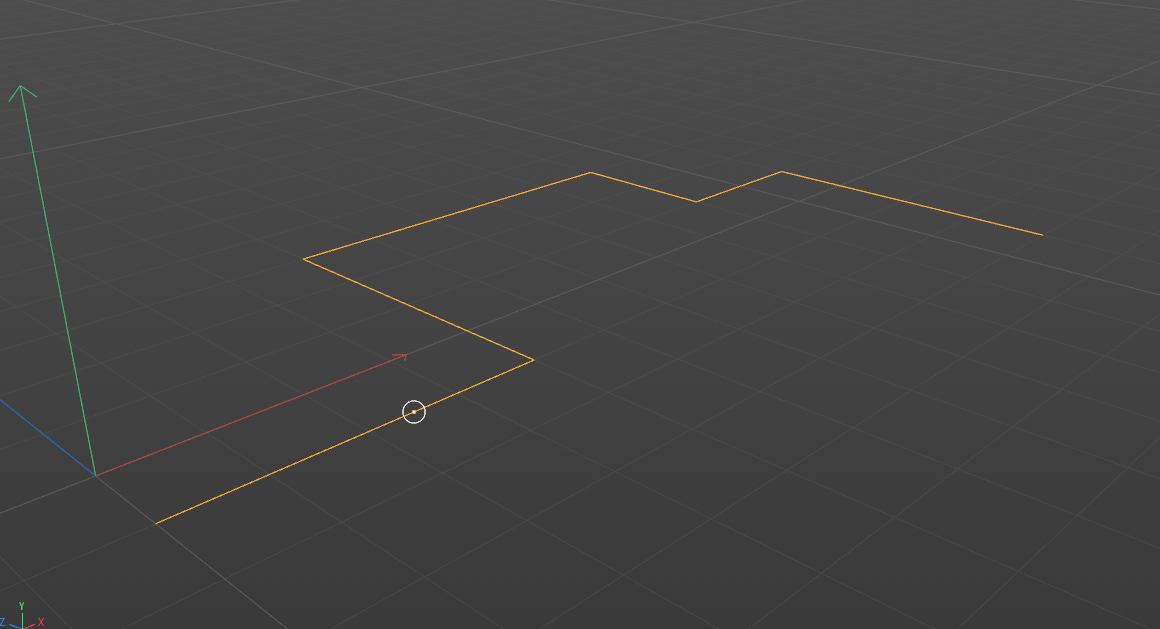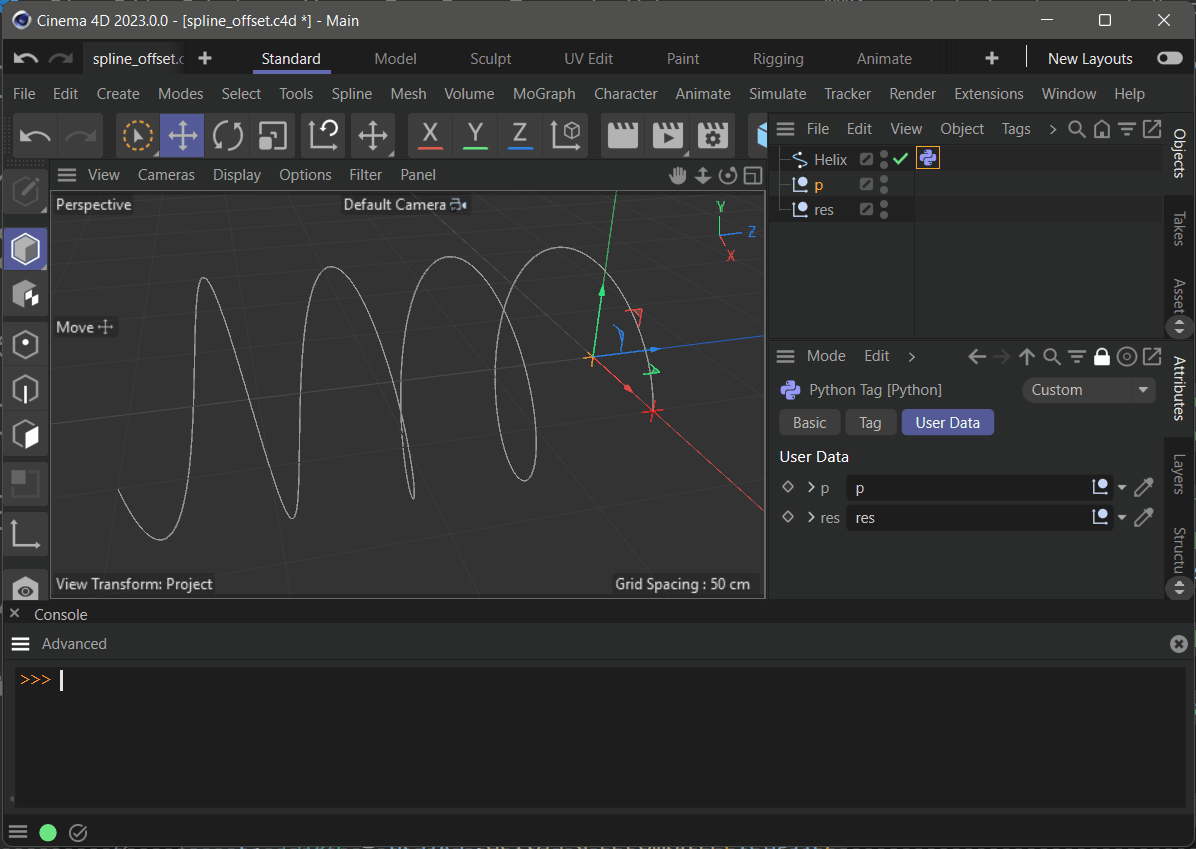# GetSplinePoint REVERSE

Happy Monday, developer friends!
I would need a way to reverse the GetSplinePoint command,

I would like, given the position of a null object, to know its value as a percentage on the spline.

Thank youHello @caleidos4d,

Thank you for reaching out to us. There is no such function in our API and you are expected to do this yourself as 'the reverse of the GetSplinePoint() command' is quite an ambiguous task. There are multiple interpretations of this task, but in its most straight forward interpretation, you would have to:

1. Get the underlying `LineObject` L of a `SplineObject` S, i.e., the discrete form of that spline where the spline has been baked into a set of line segments representing the spline at its current interpolation settings.
2. Find the closest line segment in cl to your query point p in L.
3. Compute da , the sum of the lengths of the line segments up to cl.
4. Project p onto cl to find its projection q.
5. Compute the distance db between the first point cl_a in cl and q.
6. Compute the final length length_to_q as da + db from the start of the line object to the closest point q .

There is the type `c4d.utils.SplineHelp` which provides convenience functions which can help you along the way, but it is not absolutely necessary. Find below an example solution as a python scripting tag. Please note this is indeed an example and not a final solution. I took multiple shortcuts here and there or assumed things.

You must implement the details yourself, as this is primarily a math problem and therefore out of scope of support.

Cheers,
Ferdinand

The example file: spline_offset.c4d
The result:The code of the Python Programming tag:

``````"""Demonstrates how to compute the offset for a spline at a given point in world coordinates.

The underlying problem here is that it is very unlikely that a point will sit directly on a spline.
Which is why one must compute the closest point #q on a spline #S for a given point #p. There are
different levels of complexity in which one can do this and it depends on what one actually wants
to do.

In principle, "the closest point #q on a spline #S for a given point #p" is not well defined because
a spline is just a set of polynomials, so one cannot just compute the closest point on it. One can:

a. Compute the closest point #q on the underlying line object #L of #S, i.e., the discrete form
of the spline. This is what I did and it will line up with what you see in the editor.
b. Compute an approximation of #q on #S. One could use multiple approaches to do this and I have
not done this here. This would effectively ignore the interpolation settings of a spline and
therefore not align with what one sees in the editor or a rendering.

Note:
* This is a Python Programming Tag solution which requires a specific user data setup. Please
use the also provided file.
* There are multiple levels of complexity with which this can be done. I ignored here for
example splines with more than one spline segment.
"""

import c4d
import typing

op: c4d.BaseTag # The Python scripting tag.
doc: c4d.documents.BaseDocument # The document evaluating this tag.

def main() -> None:
"""
"""
# Get the two null objects as the in- and output from the user data.
pointNull: typing.Optional[c4d.BaseObject] = op[c4d.ID_USERDATA, 1]
resultNull: typing.Optional[c4d.BaseObject] = op[c4d.ID_USERDATA, 2]
if None in (pointNull, resultNull):
return

# Get the hosting spline object, one could also deal here with spline object generators, but
# I did not. I am also ignoring the possibility that #spline could be a spline with more than
# one spline segment, and if #spline is closed or not (I always assume that it is open).
spline: c4d.SplineObject = op.GetMain()
if not isinstance(spline, c4d.SplineObject):
raise TypeError("Tag host is not an editable spline.")

# Initialize a spline helper instance and get the line object of the spline. A line object
# represents the discrete form of a smooth spline, i.e., the output of the interpolation
# settings of a spline. It is just a list of points forming line segments. It is important to
# pass SPLINEHELPFLAGS_RETAINLINEOBJECT to .InitSplineWith() when one wants to use
# GetLineObject(). One could technically also retrieve the line object from the spline itself,
# but this form is a bit more convenient.
helper: c4d.utils.SplineHelp = c4d.utils.SplineHelp()
if not helper.InitSplineWith(spline, c4d.SPLINEHELPFLAGS_RETAINLINEOBJECT):
raise RuntimeError("Could not initialize spline helper.")

line: c4d.LineObject = helper.GetLineObject()
if line is None:
raise RuntimeError("Could not access line object.")

# Get the global transform of the spline object and transform the input point #p into the local
# coordinate system of #spline. I.e., #p is now in the same coordinate system as the points of
# #spline. Also get all points of #spline and the max point index.
mgSpline: c4d.Matrix = spline.GetMg()
p: c4d.Vector = ~mgSpline * pointNull.GetMg().off
points: list[c4d.Vector] = line.GetAllPoints()
maxIndex: int = line.GetPointCount() - 1

# Compute a list of (index, distance) tuples sorted by distance for each point #q in the points
# of #spline and the input #p. #index is the index of the point #q and #distance the distance
# squared between #p and #q.
distances: list[tuple[int, float]] = [
(i, (p - q).GetLengthSquared()) for i, q in enumerate(points)]
distances.sort(key=lambda item: item)

# Get the index of the vertex #ci closest to #p. The closest point on #spline for #p lies some-
# where between #ai and #bi.
ci: int = distances
ai: int = ci if ci < maxIndex else ci - 1
bi: int = ci - 1 if ci < maxIndex else ci

# In a simplified manner, it looks something like this now:
#
# Input :                      p
# Spline:  0 -- 1 -- ... -- a -- b -- ... -- b + n
#
# #ab is the closest line segment to #p and we must first sum up the length of the line segments
# up to #ab and then add the distance between #a and the projection #q of #p onto #ab.

# Get the projection of #p onto #ab. Could also be done manually with the dot product, but
# this is a bit more convenient as we do not have to do clamp the output ourselves.
abIntersection: c4d.Vector = c4d.utils.PointLineSegmentDistance(points[ai], points[bi], p)

# Sum up the length of line segments up to #ai and then add the length of the line segment going
# from #ai to the projection of #p.
length: float = sum([(points[i] - points[i-1]).GetLength() for i in range(1, ai + 1)])
length += (points[ai] - abIntersection).GetLength()

# Convert this length into an offset value and that offset value into a position on #spline.
t: float = helper.GetOffsetFromUnit(length)
res: c4d.Vector = helper.GetPosition(t, realoffset=False)

# Print the computed data, set the result null to #res in world coordinates, and free the helper.
print (f"{ai = }, {bi = }, {length = } {t = } {res = }")
resultNull.SetMg(c4d.Matrix(off=mgSpline*res))
helper.FreeSpline()
``````

MAXON SDK Specialist
developers.maxon.net

Thanks ferdinand
I will treasure your help. Thank you very much!